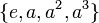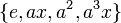# Fusion systems for dihedral group:D8

View fusion systems for particular groups | View other specific information about dihedral group:D8
There are multiple conventions on whether the term fusion system should refer to saturated fusion system or to a weaker notion. For our purposes, we will mostly be interested in saturated fusion systems, because these mimic/generalize situations where the$p$-group is a$p$-Sylow subgroup of some finite group. Unless otherwise specified, we are referring to saturated fusion systems when we talk about fusion systems.

This article discusses the possible fusion systems for the dihedral group of order eight.$G = \langle a,x \mid a^4 = x^2 = e, xax^{-1} = a^{-1} \rangle$.

There are, up to isomorphism, three possible fusion systems on$G$.

## Family contexts

Family name Parameter values General information on fusion systems for family
dihedral group (of degree, order powers of 2) degree$n = 4$, order$2n = 8$ fusion systems for dihedral groups
For more on comparison with other groups of order 8, see fusion systems for groups of order 8.

## The inner fusion system: the fusion system obtained from inner automorphisms

This is the fusion system where all morphisms are obtained as the restriction of inner automorphisms of$G$. The isomorphisms are as follows.

Equivalence class under isomorphisms Subgroups involved Order Index Total number of subgroups Are all group automorphisms of each subgroup included? Size of automorphism group from the fusion system Total number of isomorphisms (including automorphisms and others) = (number of automorphisms)$\times$$\mbox{(number of subgroups)}^2$
trivial subgroup 1 8 1 Yes 1 1$\{ e, a^2 \}$ center of dihedral group:D8 2 4 1 Yes 1 1$\{ e, x \}, \{ e, a^2x \}$ non-normal subgroups of dihedral group:D8 (some of them) 2 4 2 Yes 1 4$\{ e, ax \}, \{ e, a^3x \}$ non-normal subgroups of dihedral group:D8 (some of them) 2 4 2 Yes 1 4$\{ e, a, a^2, a^3 \}$ cyclic maximal subgroup of dihedral group:D8 4 2 1 Yes 2 2$\{ e, x, a^2, a^2x \}$ Klein four-subgroups of dihedral group:D8 (one of them) 4 2 1 No 2 2$\{ e, ax, a^2, a^3x \}$ Klein four-subgroups of dihedral group:D8 (one of them) 4 2 1 No 2 2
whole group 8 1 1 No 4 4

### Sylow subgroups realizing this fusion system

This fusion system is realized by a group having dihedral group:D8 as its 2-Sylow subgroup if and if it possesses a normal complement, so the 2-Sylow subgroup is a retract of the group and the group is a semidirect product of a normal$p'$-subgroup and the dihedral group, or equivalently the group is a 2-nilpotent group.

Some examples are below:

Group Order Isomorphism class of normal complement Is it a direct product?
direct product of D8 and Z3 24 cyclic group:Z3 Yes
dihedral group:D24 24 cyclic group:Z3 No
SmallGroup(24,8) 24 cyclic group:Z3 No
direct product of D8 and Z5 40 cyclic group:Z5 Yes
dihedral group:D40 40 cyclic group:Z5 No

## Fusion system where the center is identified with one other conjugacy class

### Explicit description of fusion system

There are in fact two versions of this viewed strictly, which are interchanged under the outer automorphism sending$x$ to$ax$ and fixing$a$.

This fusion system is realized, for instance, in the symmetric group of degree four. In fact, the symmetric group of degree four is in essence the only example -- any example admits this as a subquotient.

Equivalence class under isomorphisms, explicit description of subgroups Description of subgroups as permutation groups Subgroups involved Order Index Total number of subgroups (=1 iff weakly closed subgroup for the fusion system) Are all group automorphisms of each subgroup included? Size of automorphism group from the fusion system Total number of isomorphisms (including automorphisms and others) = (number of automorphisms)$\times$ (number of subgroups)${}^2$
trivial subgroup$()$ 1 8 1 Yes 1 1$\{ e, a^2 \}, \{ e, ax \}, \{ e, a^3x \}$$\{ (), (1,3)(2,4)\}$,$\{ (), (1,4)(2,3) \}$,$\{ (), (1,2)(3,4) \}$ center of dihedral group:D8, non-normal subgroups of dihedral group:D8 (one of the two conjugacy classes) 2 4 3 Yes 1 9$\{ e, x \}, \{ e, a^2x \}$$\{ (), (1,3) \}, \{ (), (2,4) \}$ non-normal subgroups of dihedral group:D8 (some of them) 2 4 2 Yes 1 4$\{ e, a, a^2, a^3 \}$$\{ (), (1,2,3,4), (1,3)(2,4), (1,4,3,2) \}$ cyclic maximal subgroup of dihedral group:D8 4 2 1 Yes 2 2$\{ e, x, a^2, a^2x \}$$\{ (), (1,3), (1,3)(2,4), (2,4) \}$ Klein four-subgroups of dihedral group:D8 (one of them) 4 2 1 No 2 2$\{ e, ax, a^2, a^3x \}$$\{ (), (1,4)(2,3), (1,3)(2,4), (1,2)(3,4) \}$ Klein four-subgroups of dihedral group:D8 (one of them) 4 2 1 Yes 6 6
whole group 8 1 1 No 4 4

The other version interchanges the roles of the two Klein four-subgroups, and also of the two conjugacy classes of non-normal subgroups of order two.

### Sylow subgroups realizing this fusion system

Here are some examples:

Group Order Dihedral group:D8 as a subgroup in this group Comment
symmetric group:S4 24 D8 in S4 this is the canonical, minimal example
symmetric group:S5 120 D8 in S5 the fusion system for this is precisely the same as that for D8 in S4 viewing symmetric group:S4 as an intermediate subgroup.

## Fusion system where the center is identified with both other conjugacy classes

### Explicit description of fusion system

Equivalence class under isomorphisms, explicit description of subgroups Subgroups involved Order Index Total number of subgroups (=1 iff weakly closed subgroup for the fusion system) Are all group automorphisms of each subgroup included? Size of automorphism group from the fusion system Total number of isomorphisms (including automorphisms and others) = (number of automorphisms)$\times$ (number of subgroups)${}^2$
trivial subgroup 1 8 1 Yes 1 1$\{ e, a^2 \}$,$\{ e, x \}[itex]\{ e, a^2x \}$,$\{ e, ax \}, \{ e, a^3x \}$, center of dihedral group:D8, non-normal subgroups of dihedral group:D8 (both conjugacy classes) 2 4 5 Yes 1 25$\{ e, a, a^2, a^3 \}$ cyclic maximal subgroup of dihedral group:D8 4 2 1 Yes 2 2$\{ e, x, a^2, a^2x \}$ Klein four-subgroups of dihedral group:D8 (one of them) 4 2 1 Yes 6 6$\{ e, ax, a^2, a^3x \}$ Klein four-subgroups of dihedral group:D8 (one of them) 4 2 1 Yes 6 6
whole group 8 1 1 No 4 4